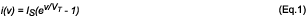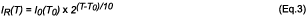# Overvoltage Protection (OVP) for Sensitive Amplifier Applications

### Abstract

This article explains the basics of reverse-biased diodes, discusses several protection strategies, and provides a few solutions for reducing parasitic leakage current and capacitance.

### Introduction

For amplifier applications that require overvoltage protection (OVP), as well as low distortion, low noise, and high bandwidth, designers must pay careful attention to overvoltage protection design. An overvoltage may be induced by human error, such as shorting the amplifier input to a higher supply voltage, or may be inherent to the application, such as a transducer that routinely produces voltages higher than the amplifier supply rails.

Most amplifier overvoltage-protection methods utilize diodes to shunt overvoltage fault current to ground or to the supply rails. These diodes exhibit capacitance and leakage current that contribute to distortion and limit bandwidth. This article explains the basics of reverse-biased diodes, discusses several protection strategies, and provides a few solutions for reducing parasitic leakage and capacitance. Operational amplifiers are used to illustrate protection methods, although many of the methods are useful for discrete amplifiers as well.

### Reverse-Biased Diode Basics

After inspecting the diode equation presented in Equation 1, one might assume that a reverse-biased diode draws a reverse current IR equal to IS.In practice, however, the reverse current is much higher than IS and is not constant across temperature and reverse-bias voltage. IR is proportional to the volume of the space-charge layer in the PN junction. Because the volume of the space-charge layer depends on applied reverse voltage, IR is typically modeled by Equation 2:where n can vary roughly from 2 to 4, depending on the manufacturer. Normally, IR vs. VR curves are found in the diode datasheet.

A widely accepted rule of thumb states that the reverse current of a PN junction doubles for every 10°C rise in temperature. Based on this rule and a reference point, we can use Equation 3 to calculate reverse current relative to temperature:I0 is the reverse current as specified at temperature T0. Usually, IR vs. T curves are found in the diode datasheet.

Equation 4 models the capacitance of a diode below its built-in potential (approximately 0.7V for silicon):Cj0 is the PN junction capacitance at 0V, Φ0 is the built-in potential, and M is the grading coefficient, which is unitless and quantifies the abruptness of the P material where it meets the N material. Equation 4 assumes VR is negative for a reverse-bias voltage and positive for a forward-bias voltage. The equation is a good model for the reverse-bias capacitance, as well as the forward-bias capacitance up to approximately half of the built-in potential. Normally, CR vs. VR curves are found in the diode datasheet.

### Basic Diode Protection

Most ICs have some form of internal electrostatic-discharge (ESD) protection. The most popular internal protection circuit employs ESD clamping diodes connected to the supply rails, thereby shunting ESD strikes to the power supplies. It can be argued that these diodes are sufficiently robust to handle an overvoltage if the current is limited through a series resistor; however, each IC is different and ESD protection topologies can vary considerably.

It is best to add external clamping diodes to the supply rails to minimize or eliminate the amount of overvoltage current that flows into an IC terminal (Figure 1).The basic diode-protection method shown in Figure 1 clamps the input voltage of the amplifier to VCC + VFBD and VEE - VFBD, where VFBD is the forward-biased voltage drop of the diode. The overvoltage current is limited by RLIMIT according to Equation 5:where VSUPPLY is VEE or VCC. This protection method also works for the inverting op-amp configuration, where RLIMIT serves as a gain-setting resistor.

The forward-voltage drop of an ordinary silicon diode is close to that of an internal ESD diode. This means that in an overvoltage condition both the internal and external diodes share the overvoltage current. Because the forward-drop matching between the two types of diodes is unknown, it is good practice to assume that all the overvoltage current will flow through the internal ESD diode. An industry rule of thumb is to select RLIMIT so that no more than 5mA will flow through the IC input.

Schottky diodes exhibit a lower forward drop (0.3V) and are frequently used in this protection topology to shunt most of the fault current. The lowest leakage Schottky diodes, however, exhibit orders of magnitude more leakage current than the lowest leakage silicon diodes. In applications where input bias currents approach nanoamperes or lower, Schottky leakage becomes overbearing. Also, a Schottky diode's forward drop can easily increase to 0.7V with temperature and forward-bias current. The popular 1N5711 Schottky diode has a maximum forward drop of 1V with 15mA of bias current at room temperature.

Protection-diode reverse-bias leakage becomes important when amplifier input bias currents are small. Ideally, the leakage currents in both protection diodes are equal and no offset is introduced. However, in practice the diodes are not perfectly matched and the leakage currents change with input voltage and temperature, thereby creating offset error and nonlinearities. A good rule of thumb is to keep the maximum reverse-leakage current ten times smaller than the input bias current of the amplifier.

Protection-diode reverse-bias capacitance, CR, is also an important design criterion to consider. This reverse-bias capacitance across each diode, combined with RLIMIT, creates a lowpass filter with a cutoff frequency, which can be calculated using Equation 6:Recall that CR is a function of the applied voltage; thus, if the input-voltage swing is large, significant nonlinearities may be introduced.

Protection-circuit overvoltage recovery time should also be considered. When a diode is forward biased, charge is stored in the PN junction's depletion region. To turn the diode off, the charge must be removed from the depletion region. Although manufacturers of high-speed switching diodes generally specify a reverse-recovery time trr, manufacturers of lower-leakage diodes generally do not. Reverse-recovery time can be measured if it is not specified in the datasheet.

Many IC companies package diode arrays that have fairly good reverse-leakage current and capacitance specifications. For example, the MAX3202E ESD protection-diode array exhibits a 1nA (max) leakage current, with 5pF of capacitance per channel. For a lower reverse-leakage current, diode-connected 2N3904s are a good choice. The PAD1 diodes by Vishay exhibit even lower reverse-leakage current and capacitance: 1pA (max) and 0.8pF (max), respectively.

### Diode Protection to Ground

Shunting overvoltage current to the supply rails means that the power supplies must absorb the current. Many power supplies cannot sink current. Shunting overvoltage current to a supply that cannot sink current is acceptable if the total load connected to the power supply is larger than the fault current or if overvoltage protection exists at the supply. If nothing within the circuit can sink the overvoltage current, the supply voltage will rise, potentially damaging components connected to the supply.A zener diode can be used as an overvoltage protector that shunts fault current to ground through a current-limiting resistor (Figure 2). Note that zener protection is only effective if the zener voltage is less than the supply voltage.

The overvoltage current is limited by RLIMIT according to Equation 7:where VFBZ is the forward drop of the zener diode and VRBZ is the reverse drop of the zener diode, both of which depend on temperature and bias current. The sum of the reverse and forward drops must be less than the supply rails so that the internal ESD diodes of the amplifier do not turn on.

In general, zener diodes exhibit higher reverse-leakage currents than regular silicon diodes. This reverse current increases dramatically near the break-over voltage and is sometimes referred to as the "knee" in I vs. V curves. If the input-signal swing is large, nonlinearities are introduced. A zener diode's capacitance also varies considerably with applied voltage and is typically much higher than a normal silicon diode.

The bandwidth and leakage characteristics of the zener protection method can be improved by connecting zeners in parallel and adding normal silicon diodes in series (Figure 3). The overvoltage current is limited by RLIMIT according to Equation 8:The result is a reduction in total capacitance such that the input signal source roughly sees only 2 × CR. This technique also reduces the leakage to roughly that of normal silicon diodes. Note that all the above diode-protection methods work in the inverting op-amp configuration as well.

### Differential Diode Protection

One of the best methods for maintaining a constant leakage current and capacitance is to keep the voltage across the protection diodes equal to 0V. The differential diode protection method keeps a 0V bias across the protection diodes during normal amplifier operation (Figure 4). During an overvoltage, the diodes conduct fault current to ground.For the inverting op-amp configuration, the overvoltage current is limited by RLIMIT according to Equation 9:For the noninverting op-amp configuration, the overvoltage current is limited by RLIMIT according to Equation 10:### Signal-Protector Integrated Circuits

Signal-protector ICs provide overvoltage-detection circuitry in combination with MOSFET switches (Figure 5).While the input signal is within the supply rails, the signal protector acts as a series resistor. When an overvoltage condition occurs, the signal protector acts as an open circuit.

There are several possible advantages to using a signal protector. First, the leakage currents are small enough for many applications; the MAX4505, for example, has a ±500pA (max) leakage current at 25°C. Second, signal protectors do not exhibit the strong relationship between input voltage and leakage current or capacitance that is inherent to passive devices. Third, when the supplies are off, the signal protector can handle ±40V at its input without any damage, while maintaining its output at 0V.

Unfortunately, the fault recovery time may be too slow for some applications. In addition, if cost is an important factor, discrete solutions may be more suitable.

### Noise Considerations

Amplifier bias currents contain noise. When this current noise flows through a resistor, a voltage noise is produced. In addition to producing noise due to amplifier bias current, a resistor produces thermal noise that is equal to, where k is Boltzmann's constant, T is the temperature in Kelvin, B is the bandwidth, and R is the resistance. The total input referred noise of an operational-amplifier circuit is given by Equation 11:Rp and Rn are the resistances looking out of the positive and negative op-amp inputs. Rn is normally equal to the gain-setting resistors in parallel (RF//RI). Vp and Vn are the voltage noises of the positive and negative op-amp inputs. Ip and In are the current noises of the positive and negative op-amp inputs. Equation 11 indicates that RLIMIT contributes to system noise by contributing to Rp or Rn (depending on the configuration). If you use zener overvoltage protection, make sure to add the noise of the zener into the equation.

A similar article appeared in two parts on December 7, 2006, and December 10, 2006, on the website Planet Analog.

### References

Frederiksen, Thomas, M. Intuitive IC Op Amps. Santa Clara, CA: National Semiconductor Corp., 1984.

Garcia, Adolfo, and Wes Freeman. "Section 7: Overvoltage Effects on Analog Integrated Circuits." Practical Analog Design Techniques. In Seminars & Webcasts—ADADC80 [database online]. Norwood, MA: Analog Devices, Inc. [cited 1 May 2007].

Gray, Paul R., Paul J. Hurst, Stephen H. Lewis, and Robert G. Meyer. Analysis and Design of Analog Integrated Circuits. New York, NY: John Wiley & Sons, Inc., 2001.

Jung, Walt, ed. Op-Amp Applications Handbook. Burlington, MA: Newnes, 2005.

Ott, Henry W. Noise Reduction Techniques in Electronic Systems. New York, NY: John Wiley & Sons, Inc., 1988.